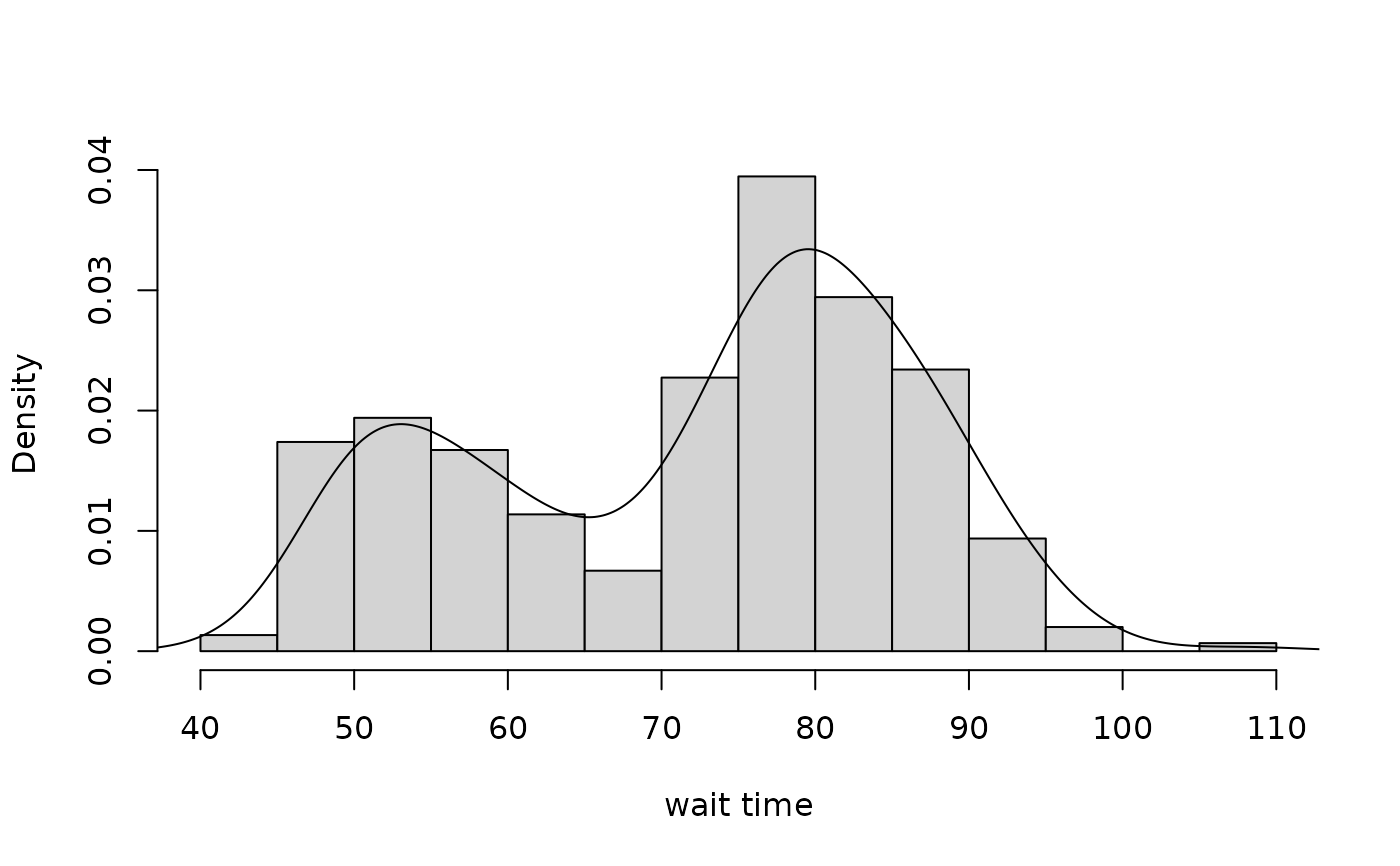Data for Exercise 5.12 and 5.111

Faithful

## Format

A data frame/tibble with 299 observations on two variables

time

a numeric vector

eruption

a factor with levels 1 and 2

## Source

A. Azzalini and A. Bowman, "A Look at Some Data on the Old Faithful Geyser," Journal of the Royal Statistical Society, Series C, 39 (1990), 357-366.

## Examples


t.test(time ~ eruption, data = Faithful)
#>
#> 	Welch Two Sample t-test
#>
#> data:  time by eruption
#> t = -25.56, df = 208.34, p-value < 2.2e-16
#> alternative hypothesis: true difference in means is not equal to 0
#> 95 percent confidence interval:
#>  -25.91029 -22.19957
#> sample estimates:
#> mean in group 1 mean in group 2
#>        57.10909        81.16402
#>
hist(Faithful$time, xlab = "wait time", main = "", freq = FALSE) lines(density(Faithful$time))if (FALSE) {
library(ggplot2)
ggplot2::ggplot(data = Faithful, aes(x = time, y = ..density..)) +
geom_histogram(binwidth = 5, fill = "pink", col = "black") +
geom_density() +
theme_bw() +
labs(x = "wait time")
}